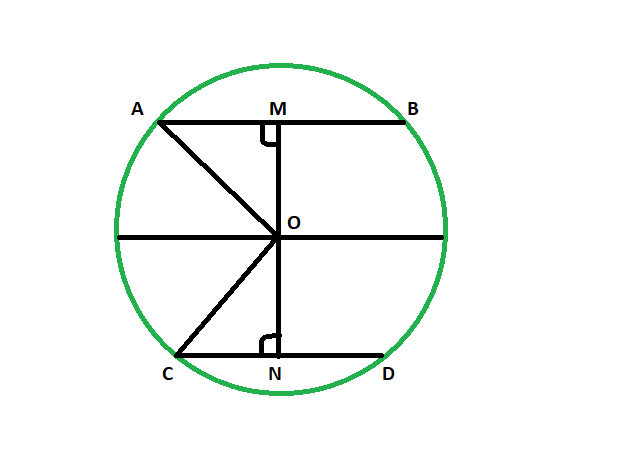Open in App
Not now

# Distance of chord from center when distance between center and another equal length chord is given

• Last Updated : 30 May, 2022

Given two equal length chords of a circle and Distance between the centre and one chord. The task is here to find the distance between the centre and the other chord.
Examples:

```Input: 48
Output: 48

Input: 82
Output: 82```Below is the implementation of the above approach:
Approach
Let AB & CD be the two equal chords of the circle having center at O.OM be the given distance of the chord AB from center.
now in triangle AOM and CON
OA = OC (radii of same circle)
MA = CN (since OM and ON are the perpendicular to the chord and it bisects the chord and AM = MB & CN = CD)
angle AMO = angle ONC = 90 degrees
so the triangles are congruent
so, OM = ON

Equal chords of a circle are equidistant from the centre of a circle.

Below is the implementation of the above approach:

## C++

 `// C++ program to find the distance of chord` `// from center when distance between center` `// and another equal length chord is given`   `#include ` `using` `namespace` `std;`   `void` `lengequichord(``int` `z)` `{` `    ``cout << ``"The distance between the "` `         ``<< ``"chord and the center is "` `         ``<< z << endl;` `}`   `// Driver code` `int` `main()` `{` `    ``int` `z = 48;` `    ``lengequichord(z);` `    ``return` `0;` `}`

## Java

 `// Java program to find the distance of chord` `// from center when distance between center` `// and another equal length chord is given/`   `import` `java.io.*;`   `class` `GFG` `{` `    `  `static` `void` `lengequichord(``int` `z)` `{` `    ``System.out.println (``"The distance between the "``+` `    ``"chord and the center is "``+ z );` `}`   `// Driver code` `public` `static` `void` `main (String[] args) ` `{`   `    ``int` `z = ``48``;` `    ``lengequichord(z);` `}` `}`   `// This code is contributed by jit_t.`

## Python 3

 `# Python 3 program to find the distance of chord` `# from center when distance between center` `# and another equal length chord is given`   `def` `lengequichord(z):` `    ``print``(``"The distance between the"` `, ` `          ``"chord and the center is"` `, z )`   `# Driver code` `if` `__name__ ``=``=` `"__main__"``:` `    ``z ``=` `48` `    ``lengequichord(z)`   `# This code is contributed ` `# by ChitraNayal`

## C#

 `// C# program to find the distance of chord ` `// from center when distance between center ` `// and another equal length chord is given` `using` `System;`   `class` `GFG ` `{ ` `    `  `    ``static` `void` `lengequichord(``int` `z) ` `    ``{ ` `        ``Console.WriteLine(``"The distance between the "``+ ` `        ``"chord and the center is "``+ z ); ` `    ``} ` `    `  `    ``// Driver code ` `    ``public` `static` `void` `Main () ` `    ``{ ` `    `  `        ``int` `z = 48; ` `        ``lengequichord(z); ` `    ``} ` `} `   `// This code is contributed by AnkitRai01 `

## Javascript

 ``

Output:

`The distance between the chord and the center is 48`

Time Complexity: O(1)

Auxiliary Space: O(1)

My Personal Notes arrow_drop_up
Related Articles